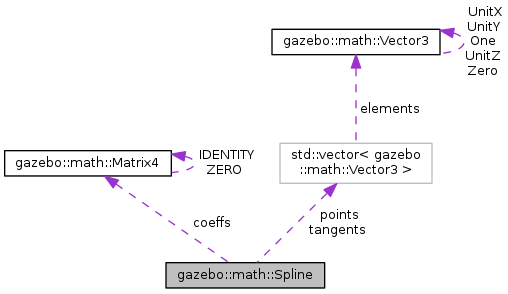##gazebo::math::Spline Class Reference

Splines. More...

`#include <math/gzmath.hh>`

Collaboration diagram for gazebo::math::Spline:[legend]

## Public Member Functions

Spline ()
constructor More...

~Spline ()
destructor More...

Adds a control point to the end of the spline. More...

void Clear ()
Clears all the points in the spline. More...

Vector3 GetPoint (unsigned int _index) const
Gets the detail of one of the control points of the spline. More...

unsigned int GetPointCount () const
Gets the number of control points in the spline. More...

Vector3 GetTangent (unsigned int _index) const
Get the tangent value for a point. More...

double GetTension () const
Get the tension value. More...

Vector3 Interpolate (double _t) const
Returns an interpolated point based on a parametric value over the whole series. More...

Vector3 Interpolate (unsigned int _fromIndex, double _t) const
Interpolates a single segment of the spline given a parametric value. More...

void RecalcTangents ()
Recalculates the tangents associated with this spline. More...

void SetAutoCalculate (bool _autoCalc)
Tells the spline whether it should automatically calculate tangents on demand as points are added. More...

void SetTension (double _t)
Set the tension parameter. More...

void UpdatePoint (unsigned int _index, const Vector3 &_value)
Updates a single point in the spline. More...

## Protected Attributes

bool autoCalc
when true, the tangents are recalculated when the control point change More...

Matrix4 coeffs
Matrix of coefficients. More...

std::vector< Vector3points
control points More...

std::vector< Vector3tangents
tangents More...

double tension
Tension of 0 = Catmull-Rom spline, otherwise a Cardinal spline. More...

Splines.

## Constructor & Destructor Documentation

 gazebo::math::Spline::Spline ( )

constructor

 gazebo::math::Spline::~Spline ( )

destructor

## Member Function Documentation

 void gazebo::math::Spline::AddPoint ( const Vector3 & _pt )

Adds a control point to the end of the spline.

Parameters
 void gazebo::math::Spline::Clear ( )

Clears all the points in the spline.

 Vector3 gazebo::math::Spline::GetPoint ( unsigned int _index ) const

Gets the detail of one of the control points of the spline.

Parameters
 [in] _index the control point index
Returns
the control point, or [0,0,0] and a message on the error stream
 unsigned int gazebo::math::Spline::GetPointCount ( ) const

Gets the number of control points in the spline.

Returns
the count
 Vector3 gazebo::math::Spline::GetTangent ( unsigned int _index ) const

Get the tangent value for a point.

Parameters
 [in] _index the control point index
 double gazebo::math::Spline::GetTension ( ) const

Get the tension value.

Returns
The value of the tension, which is between 0.0 and 1.0
 Vector3 gazebo::math::Spline::Interpolate ( double _t ) const

Returns an interpolated point based on a parametric value over the whole series.

Parameters
 [in] _t parameter (range 0 to 1)
 Vector3 gazebo::math::Spline::Interpolate ( unsigned int _fromIndex, double _t ) const

Interpolates a single segment of the spline given a parametric value.

Parameters
 [in] _fromIndex The point index to treat as t = 0. fromIndex + 1 is deemed to be t = 1 [in] _t Parametric value
 void gazebo::math::Spline::RecalcTangents ( )

Recalculates the tangents associated with this spline.

Remarks
If you tell the spline not to update on demand by calling setAutoCalculate(false) then you must call this after completing your updates to the spline points.
 void gazebo::math::Spline::SetAutoCalculate ( bool _autoCalc )

Tells the spline whether it should automatically calculate tangents on demand as points are added.

Remarks
The spline calculates tangents at each point automatically based on the input points. Normally it does this every time a point changes. However, if you have a lot of points to add in one go, you probably don't want to incur this overhead and would prefer to defer the calculation until you are finished setting all the points. You can do this by calling this method with a parameter of 'false'. Just remember to manually call the recalcTangents method when you are done.
Parameters
 [in] _autoCalc If true, tangents are calculated for you whenever a point changes. If false, you must call reclacTangents to recalculate them when it best suits.
 void gazebo::math::Spline::SetTension ( double _t )

Set the tension parameter.

A value of 0 = Catmull-Rom spline.

Parameters
 [in] _t Tension value between 0.0 and 1.0
 void gazebo::math::Spline::UpdatePoint ( unsigned int _index, const Vector3 & _value )

Updates a single point in the spline.

Remarks
an error to the error stream is printed when the index is out of bounds
Parameters
 [in] _index the control point index [in] _value the new position

## Member Data Documentation

 bool gazebo::math::Spline::autoCalc
protected

when true, the tangents are recalculated when the control point change

 Matrix4 gazebo::math::Spline::coeffs
protected

Matrix of coefficients.

 std::vector gazebo::math::Spline::points
protected

control points

 std::vector gazebo::math::Spline::tangents
protected

tangents

 double gazebo::math::Spline::tension
protected

Tension of 0 = Catmull-Rom spline, otherwise a Cardinal spline.

The documentation for this class was generated from the following file: